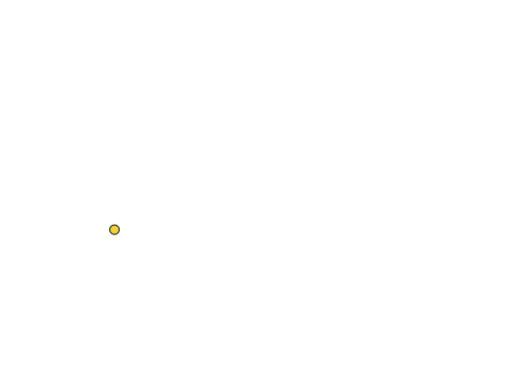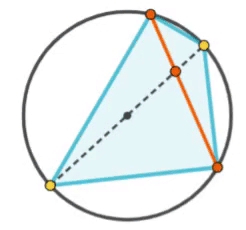Circles Collection

Concentric Circles

The length of the red segment is 2. What is the area of the blue ring?Four Arcs and a Square

All arcs are congruent. What is the area contained by the four arcs as a fraction of the square's area?Ten Circles

The orange ring is 12 pi long. What is the total length of the blue rings? Their centers lie on the orange ring's diameter.Three Circles

The red point is the center of the larger circle. What is the ratio of the yellow area to the blue area?Circle, Square, Circle

The blue quadrilateral is a square. What is the ratio of the two circles' areas?Circles vs. Squares

The two large circles have radii of 1. The dodecagon is made up of five squares. Which design contains the largest area: the four circles or the dodecagon?3 Circles or a Square?

The brown circles are congruent. The one on the left contains three identical circles, the one on the right  inscribes a square. Which design maximizes the yellow area?Two Intersecting CircleWhere should the two moving points be placed for the pink segment to be at its maximum possible length?

Determine the position of the orange chord such that the area of the blue quadrilateral is maximum.